Home > VHDL > Flipflops > DFF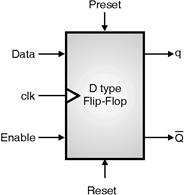D-type Flip-Flop :

* Figure shows a highly parameterized D-type flip-flop. Upon a trigger of an enabled scalar input port clk, the data from the input port data is propagated into the output ports q and qbar. The output port qbar has a bitwise inverted value of the output port q.

* The model can be triggered on the rising or the falling edge of the scalar input port clk. The polarity of clk is controlled by the enumerated parameter clk_type (Rising, Falling).

* The flip-flop has a scalar input port enable with its mode can controlled by the enumerated parameter Load_type (AsyncActiveHigh, SyncActiveHigh, AsyncActiveLow, SyncActiveLow).

* In asynchronous mode, the enable input has a higher priority over the clk input and in synchronous mode; the clk input has a higher priority over the enable input.

1) Rising (Positive) Edge Flip-Flop using if statement :

* Following example shows the behavioral VHDL code for a positive-edge-triggered D flip-flop.

•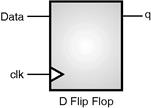Again, the code does not specify what is assigned to q when the condition in the IF statement is false, so it implies the use of a memory element.

library IEEE;

use IEEE.std_logic_1164.all;

entity dff is

port (data, clk : in std_logic;

q : out std_logic);

end dff;

architecture behav of dff is

begin

process (clk)

begin

if (clk'event and clk = '1') then

q <= data;

end if;

end process;

end behav;

2) Rising (positive) edge flip-flop using wait statement :

* A wait statement containing an edge expression causes synthesis tools to create flip-flops for all signals, and some variables are assigned values in the process. Following example shows the most common usage of the wait statement to infer a flip-flop.

* You can sometimes use wait and if statements interchangeably. If possible, use the if statement, because it provides greater control over the inferred registers.

library IEEE;

use IEEE.std_logic_1164.all;

entity dff is

port (data, clk : in std_logic;

q : out std_logic);

end dff;

architecture behav of dff is

begin

process (clk)

begin

wait until clk'event and clk = '1';

q <= data;

end process;

end behav;

3) Negative edge-triggered D flip-flop :

* Following example shows the behavioral VHDL code for a negetive-edge-triggered D flip-flop.

* The only difference here is that q follows data only at the rising edge of the clock, and it is specified here by the condition "clk' event and clk = '0'."

library IEEE;

use IEEE.std_logic_1164.all;

entity dff_neg is

port (data, clk : in std_logic;

q : out std_logic );

end dff_neg;

architecture rtl of dff_neg is

begin

process (clk) begin

if (clk'event and clk = '0') then

q <= data;

end if;

end process;

end rtl;

4) Rising edge flip-flop with asynchronous reset :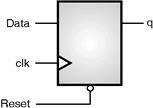library IEEE;

use IEEE.std_logic_1164.all;

entity dff_async_rst is

port (data, clk, reset : in std_logic;

q : out std_logic);

end dff_async_rst;

architecture behav of dff_async_rst is

begin

process (clk, reset)

begin

if (reset = '0') then

q <= '0';

elsif (clk'event and clk = '1') then

q <= data;

end if;

end process;

end behav;

5) Rising edge flip-flop with asynchronous reset and clock enable :

•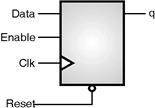The following examples infer a D type
flip-flop with an asynchronous reset and clock enable.

* In the following example, the process has been made sensitive to two reset and en. The check for the reset condition and the correct clock edge is done with a conditional.

* The check for the clock edge must include a check for activity on the clock signal using 'EVENT. This check is required since the process is sensitive to these signals.

library IEEE;

use IEEE.std_logic_1164.all;

entity dff_ck_en is

port (data, clk, reset, en : in std_logic;

q : out std_logic);

end dff_ck_en;

architecture behav of dff_ck_en is

begin

process (clk, reset) begin

if (reset = '0') then

q <= '0';

elsif (clk'event and clk = '1') then

if (en = '1') then

q <= data;

end if;

end if;

end process;

end behav;

6) Rising edge flip-flop with asynchronous preset :

The following examples infer a D flip-flop with an asynchronous preset.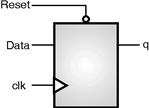library IEEE;

use IEEE.std_logic_1164.all;

entity dff_async_pre is

port (data, clk, preset : in std_logic;

q : out std_logic);

end dff_async_pre;

architecture behav of dff_async_pre is

begin

process (clk, preset)

begin

if (preset = '0') then

q <= '1';

elsif (clk'event and clk = '1') then

q <= data;

end if;

end process;

end behav;

7) Rising edge flip-flop with asynchronous reset and preset :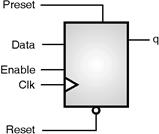• Following example shows the VHDL code for a rising edge-triggered D flip-flop asynchronous active-high preset and active-low reset inputs.

* The two asynchronous inputs are checked independently of the clock event. When either the preset or the reset input is asserted with a 1 or 0 (active), q is immediately set to 1 or 0, respectively, independent of the clock. q follows data at the rising edge of the clock; otherwise, q keeps its previous content.

library IEEE;

use IEEE.std_logic_1164.all;

entity dff_async is

port (data, clk, reset, preset, enable: in std_logic;

q : out std_logic);

end dff_async;

architecture behav of dff_async is

begin

process (clk, reset, preset)

begin

if (reset = '0') then

q <= '0';

elsif (preset = '1') then

q <= '1';

elsif (clk'event and clk = '1') then

if enable = '1' then

q <= data;

end if;

end if;

end process;

end behav;

8) Rising edge flip-flop with synchronous reset :

* The previous examples illustrate how to infer a D flip-flop with asynchronous controls one way to initialize or control the state of a sequential device. You can also synchronously reset or set the flip-flop

* When the target technology library does not have a D flip-flop with synchronous reset, synthesis tool infers a D flip-flop with synchronous reset logic as the input to the D pin of the flip-flop.

* If the reset (or set) logic is not directly in front of the D pin of the flip-flop, initialization problems can occur during gate-level simulation of the design. The following examples infer a D flip-flop with a synchronous reset.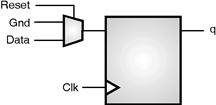library IEEE;

use IEEE.std_logic_1164.all;

entity dff_sync_rst is

port (data, clk, reset : in std_logic;

q : out std_logic);]

end dff_sync_rst;

architecture behav of dff_sync_rst is

begin

process (clk) begin

if (clk'event and clk = '1') then

if (reset = '0') then

q <= '0';

else q <= data;

end if;

end process;

end behav;

9) Rising edge flip-flop with synchronous preset :

The following examples infer a D flip-flop with a synchronous preset.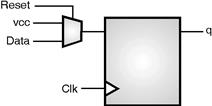library IEEE;

use IEEE.std_logic_1164.all;

entity dff_sync_pre is

port (data, clk, preset : in std_logic;

q : out std_logic);

end dff_sync_pre;

architecture behav of dff_sync_pre is

begin

process (clk) begin

if (clk'event and clk = '1') then

if (preset = '0') then

q <= '1';

else q <= data;

end if;

end if;

end process;

end behav;